## QFT Fields

These images were generated with psi.py and wave_animation.py.

### Visualizing the Fields in QFT

We often think of states in quantum field theory in terms of "particles". But what if we want to visualize QFT states directly in terms of fields?

One way to approach the problem is to look at the marginal probability density distribution of the field variable (e.g. Klein-Gordon $$\phi$$ in a scalar theory) at each point in spacetime. That is, to look at the marginal reduced densities $$\rho(\phi(x),\phi(x))$$. The practical way to do this is by approximating a scalar field with a lattice of coupled harmonic oscillators. This has been carried through nicely in simple cases by Johnson and Gutierrez 2002.

Now, in introductory QM we usually consider single-particle states which in free scalar field theory take the form $|\psi\rangle = \int dk \; \psi(k) \; a^{\dagger}_k |0\rangle .$ Defining $$\psi(x)$$ by the Fourier transform, these states will obey a relativistic Schrodinger equation $\partial_t \psi(x,t) = -i \sqrt{-\partial_x^2 + m^2} \; \psi(x,t)$ (see e.g. https://doi.org/10.1103/PhysRevD.36.2381) that reduces to the standard non-relativistic one at low energies. A typical state would be a Gaussian wavepacket wavefunction.

What do these single-particle wavepacket states look like at the field level?

### Wavefunction of a Classical Field?

Given a single particle wavepacket quantum state, what should we even expect the field picture to look like?

Here is one idea. For every real solution of $$( \partial_t^2 - \partial_x^2 + m^2) \, \varphi = 0$$ there is a unique complex solution of $$\partial_t \psi = - i \sqrt{-\partial_x^2 + m^2} \; \psi$$ such that $$\phi = {\rm Re}(\psi)$$. We can tentatively call $$\psi$$ the "classical wavefunction" for $$\phi$$.

What does this classical wavefunction look like? The following animations show the wave and wavefunction for both low and high energy normalized wavepackets. Boundary conditions are closed (clamped) at the edges. The energy density, time derivative, dispersion, and spectrum, are also plotted. Note you can read off the relativistic-ness of the behavior by comparing the spectrum and dispersion plots.

Short time scale left. Longer time scale (note phase aliasing) right. See annotation text for details.

Low Energy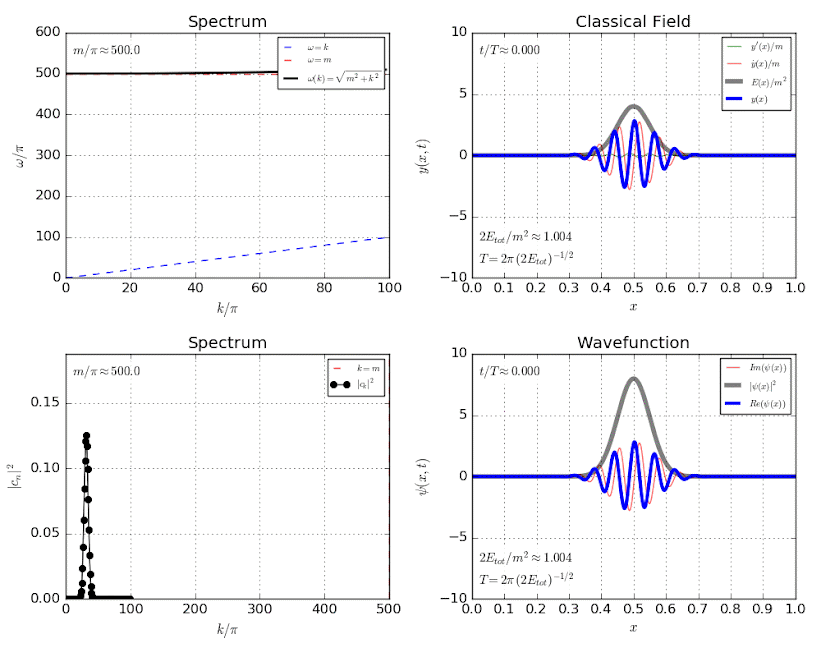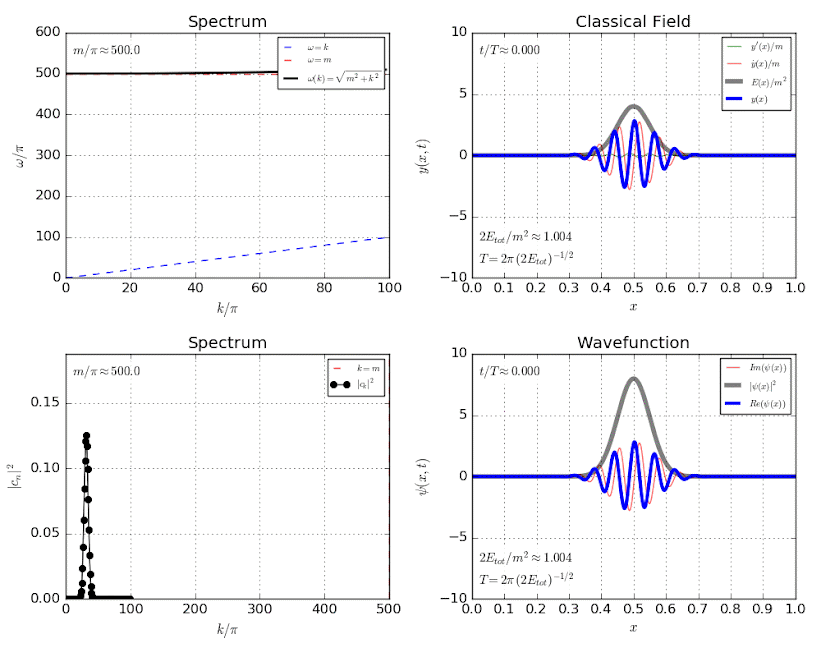Medium Energy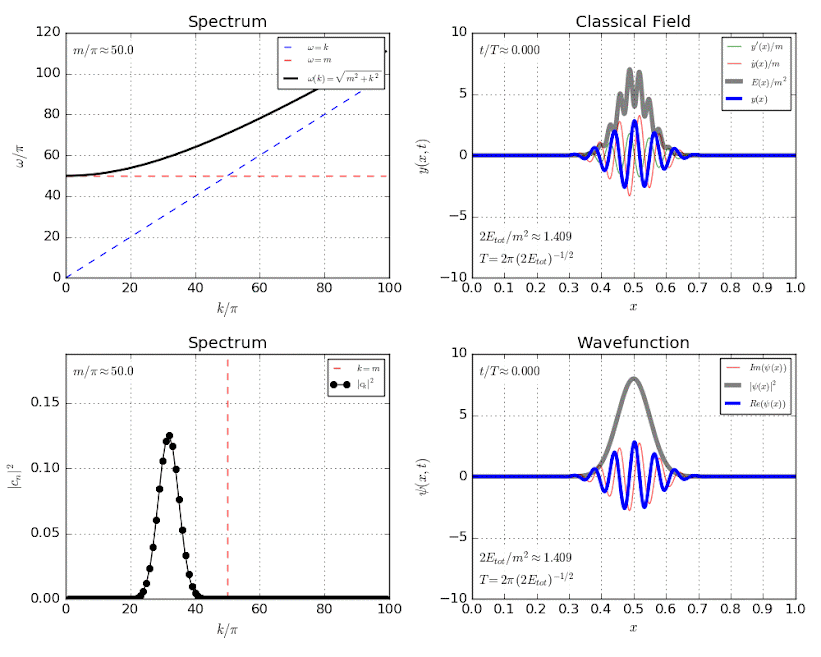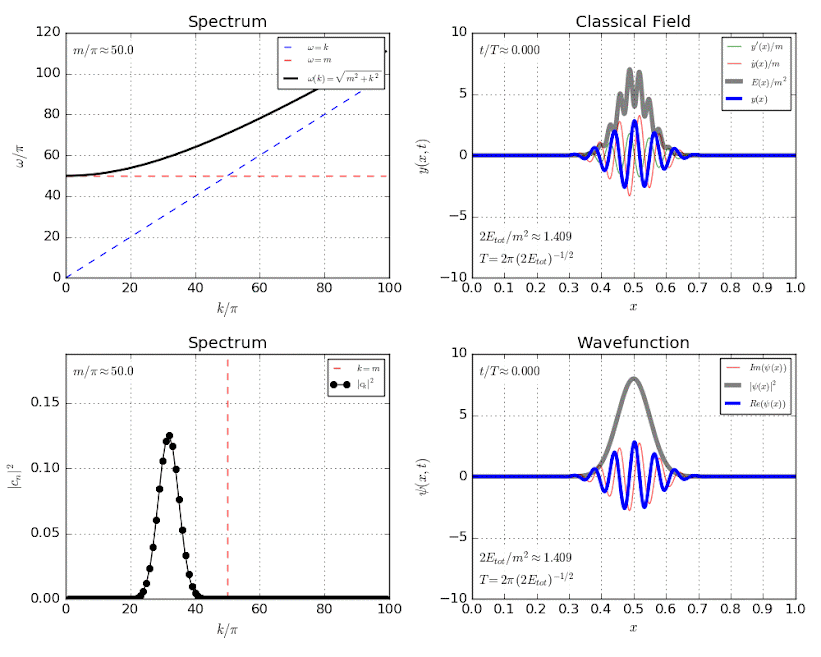High Energy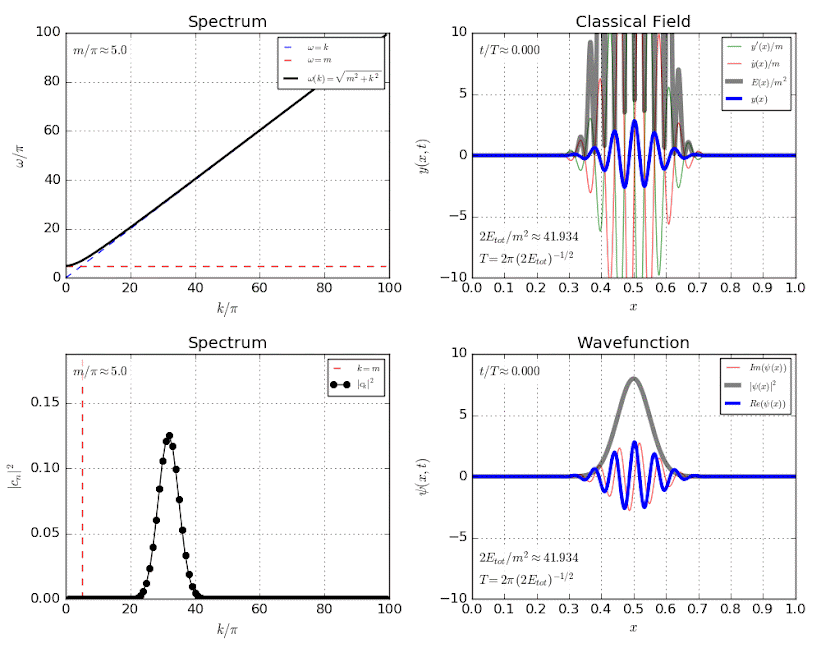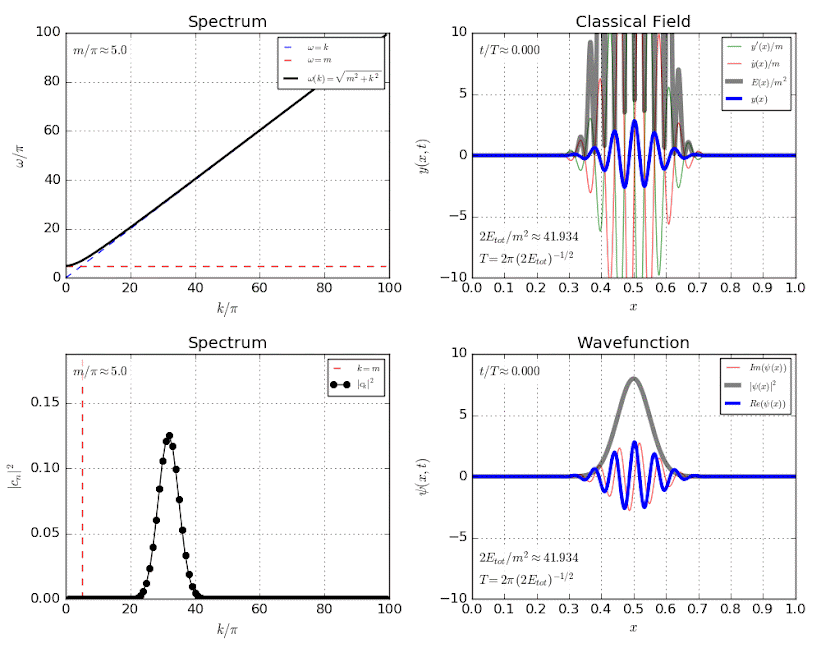### Quantum wavefunction

So does it work? Does identifying the quantum and classical wavefunctions correctly relate the classical and fields? To check, let's simplify the lattice to just a pair of coupled oscillators and look at both classical and quantum states with same "single-particle wavefunction" $$\vec{\psi}$$.

In the animation below, blue color scale shows the joint (left) and marginal (right) quantum probabilities for a single-particle state. Green dots show the classical configuration $$\vec{\phi} = {\rm Re} \vec{\psi}$$ of the oscillators. Pink dots show the magnitude squared of the oscillator wavefunction $$\vec{\psi}$$ where $$\vec{\phi} = {\rm Re} \vec{\phi}$$.

Note the key distinction between the "single-particle wavefunction" $$\psi(x)$$ (pink dots, vector) and the quantum field wavefunction $$\Psi[\phi(x)]$$ (color scale, probability).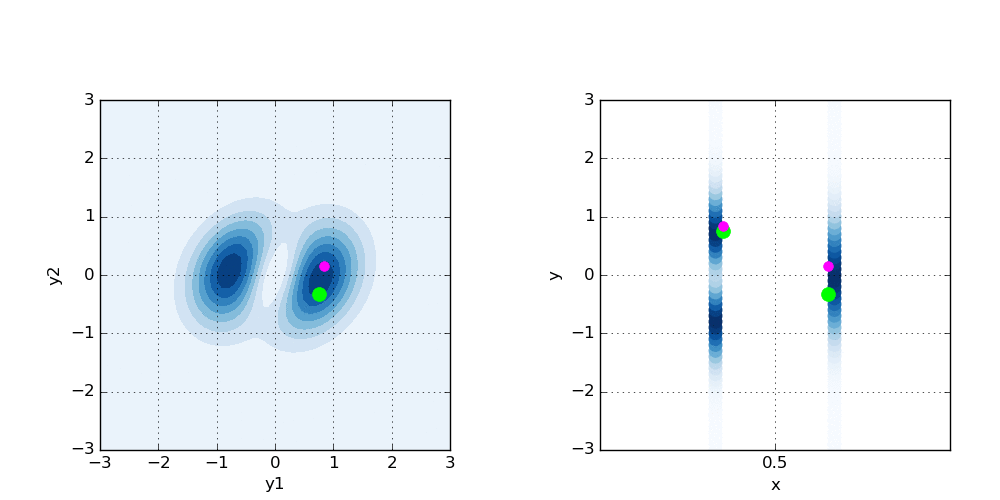There is clearly a connection, but precisely what that connection is is a little bit befuddling. Is there more can we learn from this line of questioning?